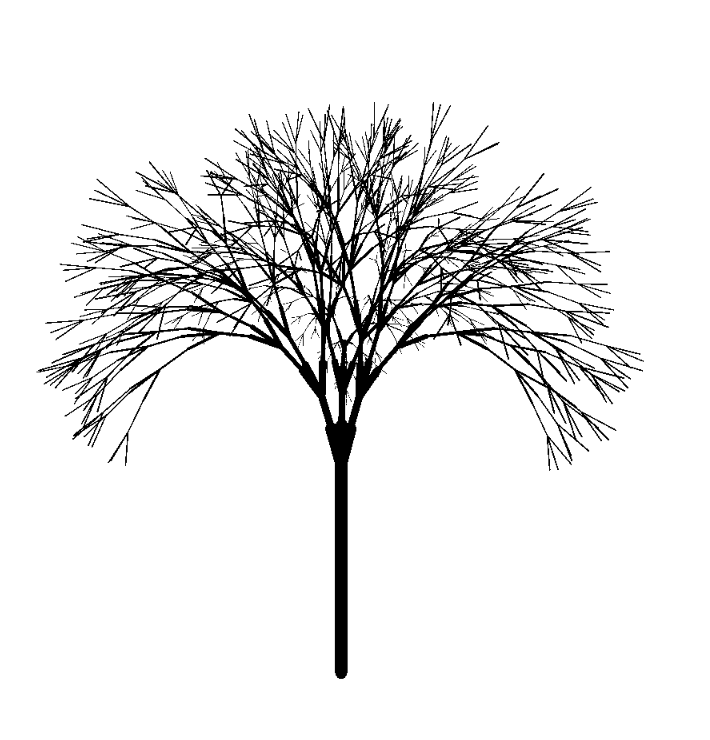### 3Parameteric L-systems

Non-terminals in L-systems can carry values that maybe updated at each step. These parameter lists are surrounded with parentheses and their elements are separated by commas, much like the mathematical notation for function application. Each parameter is written in a separate arithmetic language meaning, e.g., that the context determines if a + is rotating the turtle (when it appears outside of a parameter list) or addition (when it appears inside a parameter list).

Example:
 #lang lindenmayer racket ## axiom ## A(1,0.1) ## rules ## A(l,w) → !(w)F(l)[&(a)B(l*s,w*y)]/(d)A(l*r,w*y) B(l,w) → !(w)F(l)[-(b)\$C(l*s,w*y)]C(l*r,w*y) C(l,w) → !(w)F(l)[+(b)\$B(l*s,w*y)]B(l*r,w*y) ## variables ## n=10 r=0.9 s=0.6 a=45 b=45 d=136.75 y=0.707 w=2000 h=2000 dist=3.5 rot=0 shift=-3.2 ======================================== (provide (all-defined-out) (all-from-out lindenmayer/3d-turtle)) (require lindenmayer/3d-turtle pict3d) (define (A state variables l w) state) (define (B state variables l w) state) (define (C state variables l w) state) (define (start variables) (make-turtle (dir 0 0 (hash-ref variables 'shift)) +z +x)) (define (finish turtles variables) (define dist (hash-ref variables 'dist)) (define v (angles->dir (hash-ref variables 'rot) 0)) (define camera (basis 'camera (point-at (pos+ origin (dir-scale v dist)) (pos 0 0 .01) #:up +x))) (set-rendering-config! (hash-ref variables 'w) (hash-ref variables 'h) #:ambiance? #f) (combine camera (draw turtles (vector (rgba "brown") (rgba "saddlebrown") (rgba "chocolate")))))

Draws a black-and-white tree:In this example A, B, and C carry two parameters, l and w (which are the length and width of the line the 3d-turtle will draw).

When a Symbol has extra parameters those parameters are passed as extra arguments to the definitions below the =========.